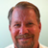# Plot x 100 bars before and after bar where calculated value is not NaN

M

#### mysti9uemirage

##### New memberHello, I'm modifying a script and based on the reversal values, I wanted to calculate fib extension values. I think my calculations are correct (in yellow). However, I want to draw a line from bar number 131 (calculated prev2Bar) to bar number 263 (barNumber + 100) for the value 821.5956, and label it (without bubbles).

As you can see in the condition below, this value will be calculated at the firstBarOfDay and latestBar. However, I want to plot that value about 100 bars before the calculated value, and 100 bars after, but nothing in between.

I can kind of draw a line by using this code (not complete but should give you an idea of what I tried):

Code:
``````#https://usethinkscript.com/threads/finding-the-first-and-last-bar-of-the-day-in-thinkorswim.526/
input Offset = 0; #hint Offset: use this to squeeze the Nth bar inwards.
def isRollover = GetYYYYMMDD() != GetYYYYMMDD();
def beforeStart = GetTime() < RegularTradingStart(GetYYYYMMDD());
def afterEnd = GetTime() > RegularTradingEnd(GetYYYYMMDD());
def firstBarOfDay = if
(beforeStart[1+offset] == 1 and beforeStart[offset] == 0) or
(isRollover[offset] and beforeStart[offset] == 0)
then 1
else 0;
def lastBarOfDay = if
(afterEnd[-1-offset] == 1 and afterEnd[offset] == 0) or
(isRollover[-1-offset] and firstBarOfDay[-1-offset])
then 1
else 0;

def prev1Up_618 = if (firstBarOfDay or isLastBar) then close + (prev1UpDiff * level_618) else double.NaN;

Plot plot_prev1Up_618 = if !IsNaN(prev1Up_618) then prev1Up_618 else
if !IsNaN(prev1Up_618) then prev1Up_618 else
if !IsNaN(prev1Up_618) then prev1Up_618 else
if !IsNaN(prev1Up_618) then prev1Up_618 else
if !IsNaN(prev1Up_618) then prev1Up_618 else
if !IsNaN(prev1Up_618) then prev1Up_618 else
if !IsNaN(prev1Up_618) then prev1Up_618 else double.NaN;``````

However, that looks really dumb, and I have to do that for a bunch of other fib lines.... there has to be a more efficient way to do this...

I think I need some kind of loop combined with some condition, so I looked into the fold operator but I'm not sure how to use it to achieve what I want. Is there any way you can show me how to plot an arbitrary number (let's say 100) for 100 bars previous to the firstBarOfDay until 100 bars after the firstBarOfDay ?

I was trying something like this, but there are syntactical errors and it has been taking me too long to tweak it to try to get the correct syntax with the logic that I want... Please help?

Code:
``plot pl_prev1Up_618 = fold index = 1 to 100 with v while (!IsNaN(prev1Up_618[i]) or !IsNaN(prev1Up_618[-i])) if !IsNaN(prev1Up_618[i]) then prev1Up_618[i] else if !IsNaN(prev1Up_618[-i])) then prev1Up_618[-i] else double.NaN;``

M

#### mysti9uemirage

##### New member
Got it working now. The idea is to have a structure that calculates and stores the offset values. This requires two def (drawCurrents, drawOffsets).
if lineLength is 10
drawCurrents: At the index where the value should be saved, it will write the following offsets: 10, 9, 8... 1
drawOffsets: Will do the look ahead for 10 values. So, for index[-10] to where ever there is value for drawCurrents (index), do calculation and stores the calculated offset: -10, -9, ... -1, 0, 1, .. 9
plot p_prev1Up_618: If there's a value for drawOffsets, GetValue based on the calculated offset

Code:
``````input lineLength = 10;

def drawCurrents = if (firstBarOfDay or isLastBar) then lineLength else
if (drawCurrents > 0 and drawCurrents <= lineLength) then drawCurrents - 1
else double.NaN;

def drawOffsets = if !IsNaN(drawCurrents[-lineLength]) then -drawCurrents[-lineLength]
else if !IsNaN(drawCurrents) then lineLength - drawCurrents
else double.NaN;
#plot p_drawOffsets = drawOffsets;

def prev1Up_618 = if IsNaN(close) then prev1Up_618 else close + 6.18;

plot p_prev1Up_618 = if IsNaN(drawOffsets) then double.NaN else GetValue(prev1Up_618, drawOffsets);``````

•Len20Referencing plot color in thinkscript Questions 9Plot ArrowDown / ArrowUp followed by AddOrder blanks out the arrows Questions 9